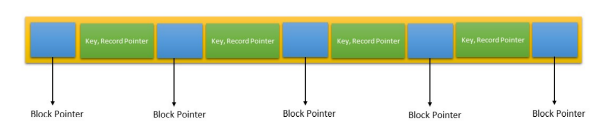# Btree

Btree is an example of multilevel indexing. Record pointers will be present at leaf nodes as well as on internal nodes.
Whereas in B+ tree we will have data (record pointers) only at leaf level.
Now we will discuss about each of the node separately:

### Root

Children: Root can have children/pointers between 2 and p inclusive.
Keys: Root can have keys between 1 to p-1 inclusive.

### Internal Node

Children: Internal Node can have children/pointers between ceil(p/2) and p inclusive.
Keys: Internal Node can have keys between ceil(p/2)-1 to p-1 inclusive.

### Leaf Node

Keys: Lead Node can have keys between ceil(p/2)-1 to p-1 keys inclusive.

Question In a B tree, suppose search key is 9 Bytes long, disk block size is 512 Bytes, record pointer is 7 Bytes, Block pointer is 6 Bytes, then calculate the order of B-tree node.
AnswerSo following above structure :
Let’s say n pointers/children we are having :
n*p + (n-1)(key_size + record pointer size) <= Block Size

So, n*6 + 5*(9+7) <= 512 n <= 24
Note 1 If we get any fraction data then take the floor of value
Note 2 If you are confused which one to take record pointer and which one to take block pointer then take the bigger one as record pointer.